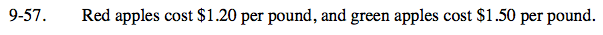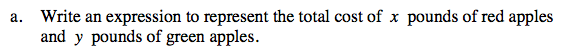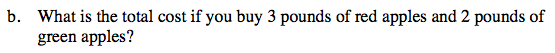Home > CC2MN > Chapter cc29 > Lesson cc29.2.1 > Problem9-57

9-57.
1. Red apples cost $1.20 per pound and green apples cost$1.50 per pound. Homework Help ✎

1. Write an expression to represent the total cost of x pounds of red apples and y pounds of green apples.

2. What is the total cost if you buy 3 pounds of red apples and 2 pounds of green apples?1.20x + 1.50ySubstitute 3 for x and 2 for y.

1.20(3) + 1.50(2) =
3.60 + 3.00 =
\$6.60